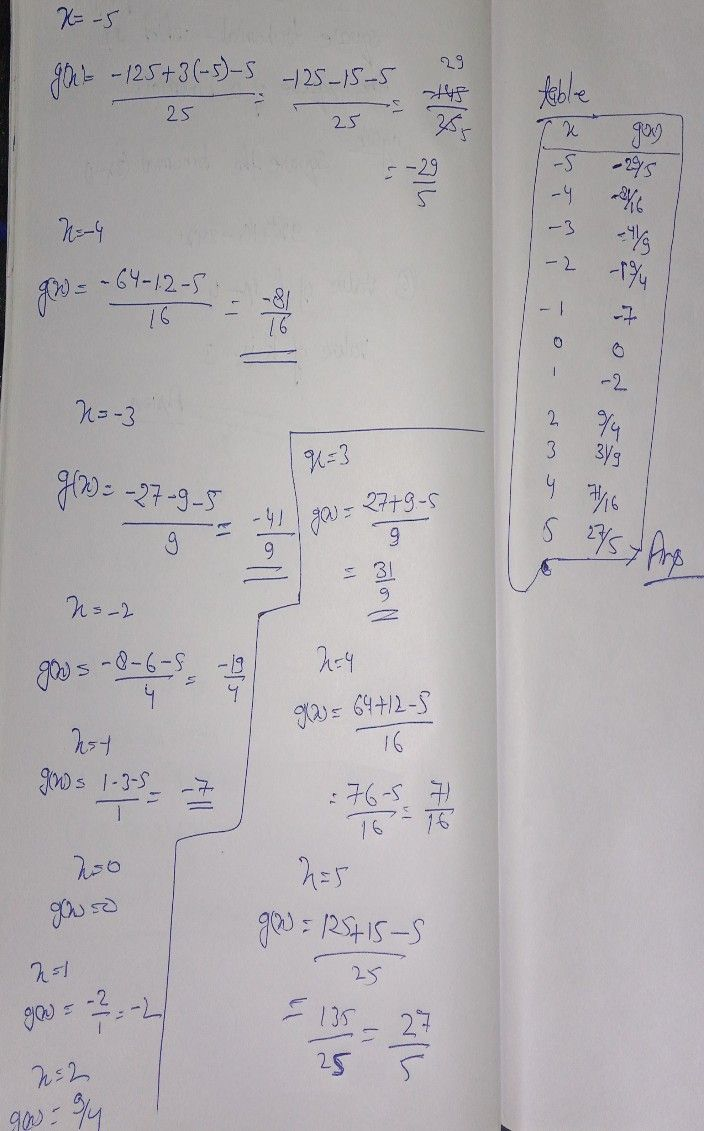Symbol
Problemthe following to enhance your learning. $1.$ Construct a table of values of the following functions using the interval of $5$ $105$ a. $g\left(x\right)=\dfrac {x^{3}+3x-5} {x^{2}}$ b. $f\left(t\right)=\dfrac {1} {t^{2-2t+1}}$ $2$ Using the data from the table of values, plot the points on the cartesian plane and connect the points of a. $g\left(x\right)$ b. $i\left(\right)$
10th-13th grade
Other
Search count: 115
SolutionQanda teacher - henryseeStudent
excuse me sir the given is g(x)=x³+3x-5/x² and also j(t)=1/t²-2t+1Qanda teacher - henrysee
yes
it is other question
you can solve the other one by understand this oneStudent
Hmm how about the number 2 sir
How to solve that number 2 problem sir??Qanda teacher - henrysee
multiple question is not allowed
you can ask me after evaluate this answer